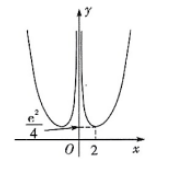$\text{A.}$ $f(x)$ 为偶函数 $\text{B.}$ $f(x)$ 的最小值为 $\frac{\mathrm{e}^2}{4}$ $\text{C.}$ 函数 $g(x)=f(x)-a\left(a>\frac{\mathrm{e}^2}{4}\right)$ 有两个零点 $\text{D.}$ 直线 $\mathrm{e} x+y-2 \mathrm{e}=0$ 是曲线 $y=f(x)$ 的切线

ABD

#### 解析：

$\mathbf{A}$ 项: 函数 $f(x)$ 的定义域为 $(-\infty, 0) \cup(0,+\infty)$, 且 $f(-x)=\frac{\mathrm{e}^{|-x|}}{(-x)^2}=\frac{\mathrm{e}^{|x|}}{x^2}=f(x)$, 故函数 $f(x)$ 为偶函数, $\mathbf{A}$ 正确;
$B$ 项: 由 $\mathrm{A}$ 项可得 $f(x)$ 为偶函数, 则 $f(x)$ 在区间 $(-\infty, 0)$ 与 $(0,+\infty)$ 的最值相等，

$\mathrm{C}$ 项: 由 $\mathrm{A}, \mathrm{B}$ 项可得, $f(x)$ 为偶函数,

$x \rightarrow+\infty$ 时, $f(x) \rightarrow+\infty$,

D 项: 当 $x>0$ 时, 设切点为 $\left(x_0, y_0\right)$,